Technical Article

# What is Dithering? Using Dithering to Eliminate Quantization Distortion

December 04, 2022 by Dr. Steve Arar

## Learn how dithering can be added to a signal to improve the performance of an analog-to-digital conversion system by eliminating quantization error and distortion.

Sometimes, electronic noise can be a blessing in disguise. In this article, we’ll take a look at “dithering,” which refers to a technique where an appropriate noise component is added to the signal to improve the performance of the A/D (analog-to-digital) conversion system.

### What is Dithering?

Most EEs are familiar with methods of limiting noise levels in electronic circuits. Filtering is a common technique that can be applied to eliminate a noise component or at least limit its bandwidth. In certain applications, such as noise-canceling headphones and noise-canceling low-noise amplifiers (LNAs), we can even measure the dominant noise component and subtract it from the system output to achieve the desired performance.

Despite these applications, there are analog-to-digital conversion systems where we need noise to improve the circuit performance. This signal processing technique, known as dithering, deliberately adds a noise signal with appropriate PDF (probability density function) and PSD (power spectral density) to the ADC (analog-to-digital converter) input (before sampling and quantization) to improve certain performance aspects of the system. Figure 1 shows the simplified block diagram of a dithered system (the diagram represents a type of dither known as non-subtractive dithering).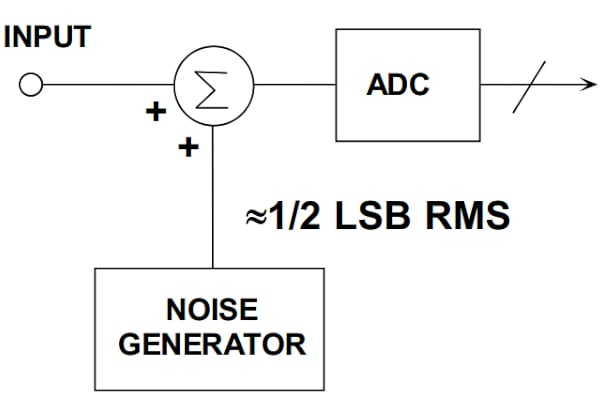##### Figure 1. Example diagram showing a block diagram for a dithered system. Image used courtesy of Analog Devices

The first time one learns about dithering, one might find it counterintuitive that some level of noise can actually be helpful in certain situations. The dithering technique can be applied for three different purposes:

1. Improving the performance of an ideal quantizer by breaking up the statistical correlation between the quantization error and the input signal
2. Randomizing the DNL (differential nonlinearity) error pattern on a non-ideal ADC to improve the spurious-free dynamic range (SFDR) performance
3. Increasing measurement resolution through averaging for slowly varying signals

In this article, we’ll discuss how dithering can improve an ideal quantizer by breaking up the statistical correlation between the quantization error and the input signal, but before that, we need to brush up on the ADC quantization noise.

### High-level Basics of ADC Quantization Error

An ADC represents a continuous range of analog values through several discrete levels, which inherently adds an error known as the quantization error. Significant research has been carried out to fully understand this error. The research history actually dates back to a 1948 paper from W. R. Bennett, "Spectra of Quantized Signals." Today, it is widely known that, under certain conditions, the quantization error can be modeled as an additive noise with a uniform distribution between $$\pm \frac{LSB}{2}$$ LSB2 (LSB denoting the least significant bit of the converter).

Also, the quantization noise is assumed to be white noise (i.e., spread uniformly over the Nyquist bandwidth dc to fs/2.) with total power equal to $$\frac{LSB^{2}}{12}$$. The flat spectrum property is based on the assumption that the quantization error samples are not correlated with one another.

We’ll refer to this model of the quantization error as the “quantization noise model” throughout this article. We’ll shortly discuss that the quantization noise model is not always valid; however, it is yet accurate enough for many practical applications. The following example shows why EEs dealing with data converters love this model!

### 10-bit vs 12-bit ADC: How Many Bits Are Enough?

Let’s consider an application where the reference voltage of the ADC is 2 V. Assume that the ADC input signal has a noise of 1 mV RMS (root-mean-square). With a 10-bit ADC, the LSB is $$\frac{2}{2^{10}}$$ = 1.95 mV, and thus, the RMS value of the noise is equal to 0.51 LSB.

From the quantization noise model, we know that the quantization operation adds an RMS noise of $$\frac{LSB}{\sqrt{12}}$$ = 0.29 LSB.

As you can see, the quantization noise is comparable with the original noise coming from the input. To find the total noise power of the system, we should add the power of the two noise sources together:

$P_{Noise, \text{ }total}=P_{input}+P_{quantization}=(0.51 \text{ }LSB)^2+(0.29 \text{ }LSB)^2=0.34 \text{ } LSB^2$

Taking the square root of this value yields the RMS of the total noise as 0.59 LSB. If this noise level is not acceptable for our application, we can increase the ADC resolution to reduce the quantization noise. For example, with a 12-bit ADC, the input noise is 2.05 LSB RMS. Compared with the input noise, the quantization noise (0.29 LSB) is now almost negligible. The total noise RMS works out to 2.07 LSB for this example. A 12-bit system seems to provide enough resolution for this application.

Having the total noise present in our signal, we can determine the signal-to-noise ratio (SNR) in an AC application or the minimum detectable signal in a measurement application. The important point here is that the noise model allows us to easily consider the effect of the quantization process on the noise performance of the system.

As a side note, it’s worthwhile to mention that the above discussion implicitly assumes that the dominant noise added by the ADC is the quantization noise. This is not always the case. As we increase the ADC resolution, the quantization noise becomes smaller and smaller. At some point, the quantization noise becomes negligible in comparison with the electronic noise within the ADC that is produced by the thermal noise and flicker noise of the ADC internal circuitry. This is the case with today’s high-resolution ΔΣ (delta-sigma) ADCs. If the quantization noise is negligible, the peak-to-peak input-referred noise of the ADC should be considered to analyze the system noise performance.

### Frequency Content of the Quantization Error

One implication of the quantization noise model is that the error is not correlated with the input. To better understand this, consider the waveforms in Figure 2.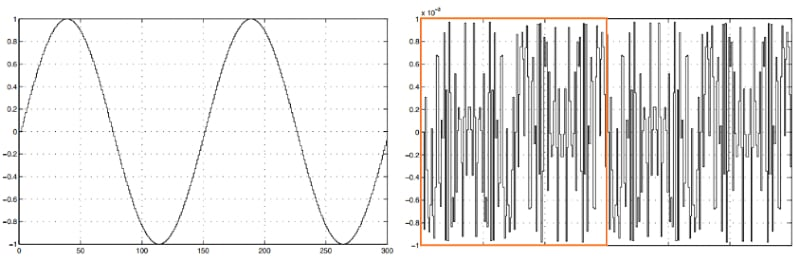##### Figure 2. Example waveforms. Image used courtesy of Franco Maloberti

The left curve in the above figure depicts two periods of a 10-bit quantized sine wave. The right curve shows the quantization error. In this example, the ratio of the sampling frequency to the input frequency is 150. You can confirm by visual inspection that the quantization error is periodic (one period is indicated by the orange rectangle). Additionally, there is a correlation between the input and the quantization error signal. From this, we know that the frequency content of a periodic signal concentrates at multiples of the fundamental frequency of the signal. This means that while the quantization noise model expects the error to have a flat frequency spectrum, the quantization error has some strong frequency components.

This is a general issue: if the input is a sinusoid and the sampling frequency is a multiple of the input frequency, the quantization error is correlated with the input signal. Another example is shown in Figure 3.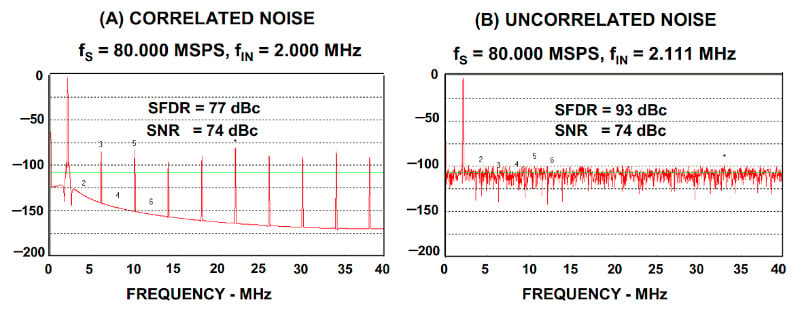##### Figure 3. Example graphs showing correlated noise (a) and uncorrelated noise (b). Image used courtesy of Analog Devices

The left curve shows the spectrum of an ideal 12-bit ADC when the input is a 2 MHz sinusoid and the sampling frequency is 80 MSPS. The right curve shows the spectrum of the same ADC for a 2.111 MHz sinusoid sampled at the same sampling frequency. As expected, different harmonics of the input frequency are produced at the output when the ratio of the sampling frequency to the input frequency is a whole number. For the left-hand curve, the spurious free dynamic range (SFDR) of the system is only 77 dBc. By slightly changing the input frequency, the harmonic components disappear, and we get a grassy-looking noise floor.

Note that the RMS value of the quantization error is the same for both cases, leading to an SNR of 74 dBc (the theoretical value obtainable by a 12-bit ADC). For both cases, the RMS error is consistent with the value predicted by the quantization noise model $$(\frac{LSB}{\sqrt{12}})$$; however, the frequency spectrum of the error is not flat in the left-hand diagram.

The above harmonic components are an artifact of the quantization process and are not related to the performance of the ADC circuitry. This highlights an important caveat about ADC testing: the spectrum we get for a single-tone sine wave fast Fourier transform (FFT) testing will be affected by the quantization process artifacts if the input signal is an exact submultiple of the sampling frequency.

To summarize, if the quantization error is correlated with the input, we cannot assume that the ADC only increases the noise floor of the input. In this case, the quantization noise model is no longer valid, and the quantization process can produce significant harmonic components in the output spectrum. Normally, we prefer the error energy to spread over a wide frequency band rather than concentrate at some specific frequencies.

### Quantizing Low-amplitude Signals

Quantizing low-amplitude signals can also lead to a correlation between the quantization error and input. An example application where low-amplitude signals can be a problem is digital audio systems. Assume that the amplitude of the ADC input drops to 0.75 LSB, as shown in Figure 4.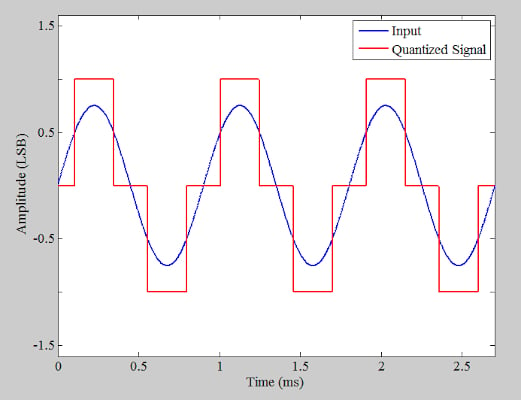##### Figure 4. Example graph showing the amplitude of the ADC input dropping.

As you can see, the quantized signal takes only three different values and has a square wave-like shape. We know that the spectrum of a square wave contains different harmonics of the fundamental frequency. In the above example, the input is a 1.11 kHz sinusoid, and the sampling frequency is 400 kHz (deliberately chosen to be much higher than that required by Nyquist's sampling theorem). The FFT of the output is shown in Figure 5.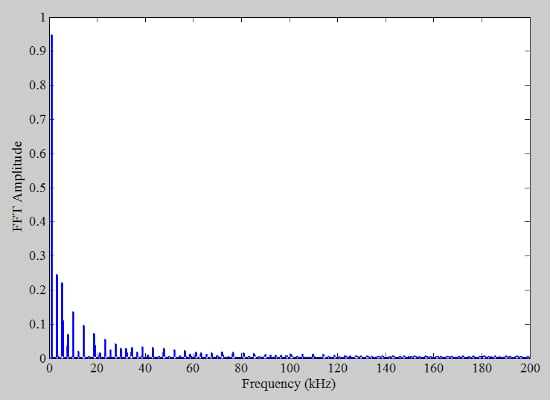##### Figure 5. Graph showing the FFT amplitude vs. frequency.

Although the input frequency (1.11 kHz) is not a submultiple of the sampling frequency (400 kHz), the spectrum contains significant harmonic components. These harmonics are more easily discernible in the zoomed-in version of the spectrum provided in Figure 6.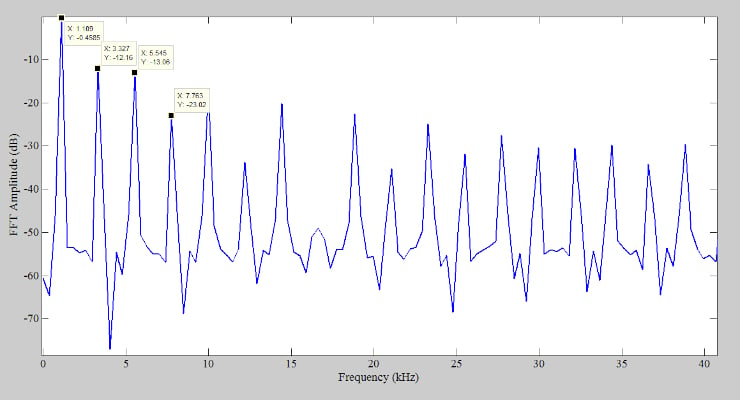##### Figure 6. A zoomed-in version of the spectrum.

To examine the dithering technique, we add noise with triangular distribution to the above signal and then quantize it. The width of the triangular dither pdf (probability density function) is taken to be 2 LSB. The waveforms are shown in Figure 7.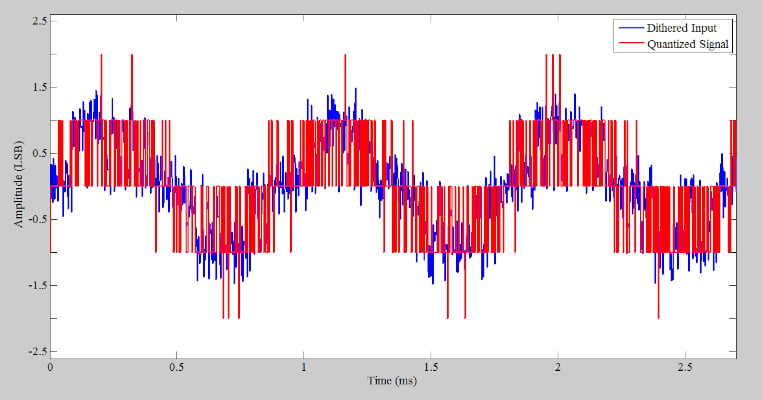##### Figure 7. Example waveforms after adding noise with triangular distribution and being quantized.

In the time domain, it seems that the information is lost, but what about the frequency domain? The spectrum of the new quantized signal (red curve above) is shown in Figure 8.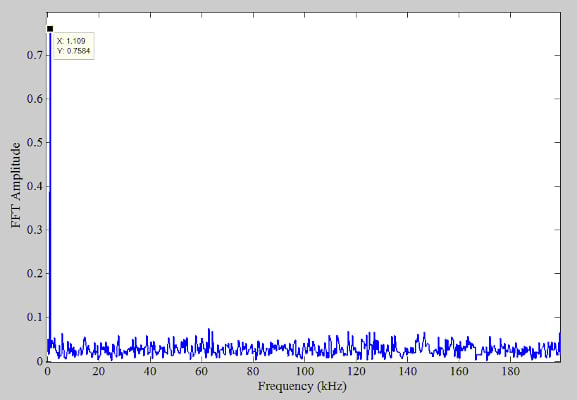##### Figure 8. The spectrum of the new quantized signal.

Dithering eliminates the harmonic components. In fact, the energy of the harmonic components is spread over a wide frequency band. As a result, we expect the noise floor to rise slightly when we apply the dithering technique. In addition to this effect, the dither noise added to the input also contributes to the increase in the noise floor.

The above example clearly shows the advantage of dithering in spectral analysis applications. However, it’s interesting to note that even without transforming the signal to the frequency domain, we can benefit from dithering. For example, in digital audio, the increase in the featureless background noise (due to dithering) is perceptually far more acceptable than the artificial harmonics introduced by the quantizer.

### Benefiting From Dithering Noise

One implication of the quantization noise model is that the quantization error is not correlated with the input. When this is not the case, the quantization operation introduces a type of distortion sometimes referred to as the “quantization distortion.” By adding the dither noise, the correlation between the quantization error and the input is eliminated. This consequently eliminates the harmonic components produced by the quantization operation. In this way, dithering can improve the performance of an ideal quantizer. As mentioned above, dithering is also applied for several other purposes. In the next article in this series, we’ll dive further into this discussion.

As a final note, it’s worth mentioning that in most systems, the input signal has enough noise, so adding additional dither noise to break the correlation between the quantization noise and input is not required. Also, the input-referred noise of the ADC might be enough to produce the same dithering effect.

Featured image used courtesy of Adobe Stock